Module 3.1 Notes "Introduction to Multiple Regression"

 Index to Module Three Notes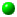3.1: Introduction to Multiple Regression3.2: Curvature, Dummy Variables and Interaction3.3: Model Building Process

Multiple regression and correlation analysis is similar to simple linear regression and correlation in that it involves the study of the form, direction and strength of relationships. But with multiple regression, the relationship is between the dependent variable and multiple independent variables rather than just one independent variable.

The seven steps we used to study simple linear regression in Module 2 will be used for multiple regression. They are repeated here for convenience.

1. Hypothesize regression model
E(Y) = B0 + B1 X1 + B2X12 + B3X2 + B4X1X2

2. Gather data and examine relationships with graphs (scatter diagrams)
3. Estimate regression model parameters
4. Test practical utility of regression model
5. Test statistical utility of regression model and multiple independent terms
6. Evaluation assumptions of regression model
7. Use model for prediction

Note that the first step shows the addition of another independent variable, X2. That variable could be another numerical variable or a categorical variable used to stratify the data. Examples of categorical or qualitative variables include male/female, in-season/out of season, and defective/not defective. The first step also shows that X1 has been raised to the 2nd power. This let's us examine upward or downward curvature in the data. The final term that has been added is a mathematical way of capturing a concept called interaction. Interaction allows us to model situations where the relationship between Y and X1 is said to depend on X2.

The objective of Module 3 is to introduce model building skills that will enable you to model relationships involving multiple numerical and categorical variables, curvature and interaction. Module 3.2 Notes will cover incorporating categorical variables, curvature and interaction separately. Model 3.3 Notes will cover model-building skills that will hopefully put everything together.

The purpose of Module 3.1 Notes is to introduce multiple regression by the addition of just one more numerical independent variable. Module Notes 3.1, 3.2 and 3.3 are designed to prepare you for Assignment 3. These note sets relate to optional readings in Chapter 14 of the Levine reference and Chapter 13 in the Mason reference given at the end of the note sets.

Step 1: Hypothesize the regression model

Equation 3.1.1 illustrates a model similar to that used in Module 2; a model to predict the external hours of time needed to audit a client based on client assets and the years of experience of the audit leader (abbreviated Exp in this illustration). My idea, or hypothesis, is that as client assets increase, external hours increase at a constant rate (positive linear relationship); and as the years of experience of the audit leader increase, external hours decrease at a constant rate (negative linear relationship).

Eq. 3.1.1: E(Y) = B0 + B1X1 - B2X2; or
E(ExtHours) = B0 + B1(Assets) - B2(Exp)

Caution

When we add a second (or many) independent numerical variable(s), we want to add independent
variables that correlate strongly with the dependent variable, but weakly with the other independent variables already in the model. As a rule of thumb, I like to make sure the correlation coefficient between any of the pairs of independent variables is less than +0.7 and greater than -0.7. If two or more independent variables are strongly related to each other, we get what's called multicollinearity. This condition makes it difficult to determine the contribution of the individual independent variables, and also adversely affects model interpretation.

Step 2: Gather Data

When you are exploring whether or not independent variables will contribute to effective predictive models, you may wish to run separate scatter diagrams between Y and each of the independent variables, and determine the correlation between Y and each of the independent variables. Here is the data I have selected for this example. Assets are in \$000s, as in Module 2; Exp (Experience of the audit team leader) is in years; and ExtHours (external hours to perform the audit) are in hours. Assets and Experience are the independent variables being used to predict External Hours, the dependent variable.

Worksheet 3.1.1

 Assets Exp ExtHours 3200 8 700 3000 7 900 3500 9 800 4000 6 900 4700 8 880 5000 6 850 6000 5 1000 5500 6 1000 6500 7 950 6500 4 1100 7000 5 1200 4500 9 830 7500 3 1100 5750 6 1100 7250 5 1160 8000 8 1120 8000 4 1300 8500 4 1400 9000 7 1500 8500 2 1200

Next, I ran the scatter diagrams relating External Hours to Assets (Worksheet 3.1.2), and External Hours to Experience (Worksheet 3.1.3).

Worksheet 3.1.2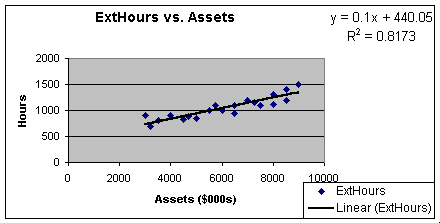Worksheet 3.1.3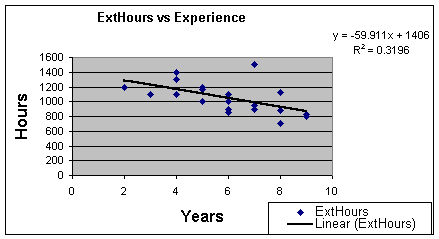Worksheet 3.1.2 shows the moderately strong positive linear relationship between External Hours and Assets. Worksheet 3.1.3 does show a negative linear relationship, but not as strong as we would like. Remember that we use the correlation coefficient, r, to measure strength of the linear relationship between two variables. While we can take the square root of the coefficients of determination, there is an Excel Data Analysis Add-In that lets us create all of the pair-wise correlation coefficients at once. Worksheet 3.3.4 shows the result. To get the correlation matrix, select Tools from the Standard Toolbar, Data Analysis from the pulldown menu, Correlation, then respond to the dialog box entry questions. If the data in Worksheet 3.1.1 above started in Column A, Row 1, and extended through Column C, Row 21, the input range for the first dialog box is A1:C21. I remember to check the Labels circle, then place the output near my data, such as D1. Click OK and Worksheet 3.1.4 shows the results:

Worksheet 3.1.4

 Assets Exp ExtHours Assets 1 Exp -0.605951 1 ExtHours 0.90407 -0.565325 1

Note the less than moderate relationship between the two independent variables, Assets and Experience (-0.606): that's good. But also note the fairly weak relationship between the dependent variable, External Hours, and Experience (-0.565): that's not so good if we want to improve our prediction of External Hours.

Model builders like to use the principle of parsimony or keeping models as simple as possible (I've heard this called kis - keep it simple, but prefer the more academic term!). When we build multiple regression models, we do run the risk of making something too complicated to interpret and understand, especially when the addition of a variable does not contribute to the prediction of the dependent variable. I want to continue with the addition of a fairly weak variable to illustrate this point.

Pause and Reflect

We are introducing a subject that involves building bigger models than the simple linear regression model. However, we need to be careful that we use good science in building these bigger models. Just because a model is bigger doesn't make it better - sometimes it's just bigger.

Step 3: Estimate the Regression Parameters

To estimate the regression parameters and generate all of the regression statistics and graphs, use the Regression Data Analysis Add In as we did in Module 2. Select Tools from the Standard Toolbar, Data Analysis from the pulldown menu, Regression, and then respond to the dialog questions as you did in building a simple linear regression model. The difference is that the X range will include highlighting all of the columns containing the X variables rather than just one column. Caution: when you load data, be sure to put all of the X variables in contiguous columns to allow their selection at one time (Note Worksheet 3.1.1 shows the two independent variables in adjacent columns). Worksheet 3.1.5 illustrates the Regression Summary.

Worksheet 3.1.5

 SUMMARY OUTPUT Regression Statistics Multiple R 0.904337925 R Square 0.817827082 Adjusted R Square 0.796394974 Standard Error 93.69957022 Observations 20 ANOVA Df SS MS F Significance F Regression 2 670041.6392 335020.8196 38.15897 5.18E-07 Residual 17 149253.3608 8779.609459 Total 19 819295 Coefficients Standard Error t Stat P-value Lower 95% Upper 95% Intercept 468.7842299 153.5922957 3.052133753 0.007206 144.7324 792.8361 Assets 0.098138845 0.014392674 6.818666744 2.99E-06 0.067773 0.128505 Exp -2.931174966 13.79061295 -0.212548563 0.834207 -32.02687 26.16452 RESIDUAL OUTPUT PROBABILITY OUTPUT Observation Predicted ExtHours Residuals Standard Residuals Percentile ExtHours 1 759.3791346 -59.37913462 -0.669959585 2.5 700 2 742.6825406 157.3174594 1.774972649 7.5 800 3 785.8896132 14.1103868 0.159203884 12.5 830 4 843.7525607 56.24743933 0.634625468 17.5 850 5 906.5874023 -26.58740234 -0.299978859 22.5 880 6 941.8914058 -91.89140582 -1.036787223 27.5 900 7 1042.961426 -42.96142593 -0.484722778 32.5 900 8 990.9608284 9.039171611 0.101986661 37.5 950 9 1086.168499 -136.1684986 -1.536354334 42.5 1000 10 1094.962023 5.037976531 0.0568422 47.5 1000 11 1141.100271 58.89972892 0.664550573 52.5 1100 12 884.0284583 -54.02845834 -0.609589273 57.5 1100 13 1196.032044 -96.03204358 -1.08350498 62.5 1100 14 1015.49554 84.50446032 0.953442207 67.5 1120 15 1165.634982 -5.634982363 -0.063578064 72.5 1160 16 1230.445591 -110.4455913 -1.246129352 77.5 1200 17 1242.170291 57.82970881 0.65247781 82.5 1200 18 1291.239714 108.7602862 1.227114486 87.5 1300 19 1331.515611 168.4843886 1.900966253 92.5 1400 20 1297.102064 -97.1020637 -1.095577743 97.5 1500

Look in the intercept, Assets and Experience Rows, in the Coefficients Column, to obtain the regression coefficients. These are repeated in Equation 3.1.2.

Eq. 3.1.2: ExtHours = 468.8 + 0.10 (Assets) - 2.9 (Experience)

The intercept, 468.8, is interpreted as in simple linear regression. External hours equal 468.8 when assets and experience equal to zero. Since we did not have any observations where assets and experience equal zero, there would be no practical interpretation.

The slope of 0.10 for assets means that external hours increase by 0.10 when assets increase by one (\$1,000 since assets are in \$000s), holding experience constant (that is, for interpretations involving team leaders with similar experience). The slope of -2.9 means that external hours decrease by 2.9 when experience increases by 1 year, holding assets constant (at similar levels).

Step 4: Test Practical Utility of Regression Model

As with simple linear regression, we use R2 and Standard Error of the Model to test practical utility of the model.

Coefficient of Multiple Determination, Adjusted R2
The R2 in multiple regression is called the coefficient of multiple determination. It is safest to use the Adjusted R2 (called Adjusted R Square in Excel), as this statistic adjusts for the number of independent variables in relation to sample size to prevent overestimating the amount of variability explained by the independent variables. A rule of thumb in multiple regression is that we should have at least 10 observations for each regression parameter. This model has three regression parameters (the intercept B0 and the two slopes, B1 and B2) so we should have 30 observations. We only have 20 so the adjusted R2 is a bit lower than the R2 - sort of like keeping us honest. The formula used by Excel to compute the adjusted R2 is:

Eq. 3.1.3: R2adjusted = 1 - { ( 1 - R2) * [ (n - 1) / (n - 2) ] }

From this formula, you can see that if the sample size was 1,000, the difference between adjusted R Square and R Square is negligible.

The adjusted R Square is found in the Regression Statistics near the top of Worksheet 3.1.5. It is 0.796. The interpretation: assets and experience explain 79.6% of the sample variation in external hours. As in simple linear regression, we would like this to be as close to 100% as possible. A rule of thumb benchmark might be at least above 50%. If you are comparing two models, the model that is most practically useful, if everything else is equal, is the model with the highest adjusted R2.

Standard Error of the Model
The Standard Error of the Model or Estimate is found directly below the adjusted R2. It is 93.7. The interpretation: we would expect 95% (or most) of the actual values of external hours to be within +/- 2 * 93.7 of the predicted external hours. As with simple linear regression, we want the standard error to be as low as possible. A benchmark might be the standard error (93.7) divided by an actual values of Y (external hours) should be lower than ranges between .05 and 0.15, or 5% to 15%. If you are given two models, the model with the lowest standard error, if everything else is equal, is the model with the most practical utility.

Pause and Reflect

Although testing practical utility involves benchmarks and judgment, it is very important. Models that are not judged practically useful should not be used, even if they are proven to be statistically significant or useful. A model could have a very steep slope and thus be statistically significant, but it might have too much error around the regression line to be of practical use.

Step 4: Test Statistical Utility

There are multiple hypothesis tests in multiple regression because not only can we test for statistical utility of the model, but we can also test for statistical utility of the multiple independent variables.

Is the Model Statistically Significant or Useful?
The null and alternative hypotheses to test for model statistical utility are:

H0: B1 = B2 = 0 (the model is not statistically useful)
Ha: At least 1 B is =/= 0 (the model is statistically useful)
(remember, =/= is the closest I can come to the "not equal" symbol)

The test statistic for this test of hypothesis is the F, which has a value of 38.16 (look in the Regression row of the ANOVA table in Worksheet 3.1.5, under the F Column). Recall, in concept, the F measures the ratio of variation explained by the regression model to the variation unexplained. The farther this number is from 1, the more variation is explained related to unexplained. The p-value for the F is 5.28E-07, or 0.000000528 (look in the Regression row of the ANOVA table in Worksheet 3.1.5,under the Significance F column). Since the p-value of 5.28E-07 is less than an alpha of 0.01, we reject the null hypothesis and conclude the model is statistically useful.

Attention to Detail

Did you notice that I used an alpha of 0.01, whereas I have been using convention of an alpha of 0.05 before now? We are entering into a new hypothesis test procedure in multiple regression where we do multiple hypothesis tests on the same data (with this example, we could actually do three tests: a model test, a test on X1, and a test on X2). Each test with the same data theoretically increases the error of rejecting a true null hypothesis. So, research convention suggests that we lower the alpha to 0.01 when we do multiple tests with the same data.

Are Assets Statistically Significant or Useful?
We can also test for statistical significance of the individual independent variables. The null and alternative hypotheses to test for the importance of assets are:

H0: B1 = 0 (assets are not important or not statistically useful)
Ha: B1 =/= 0 (assets are important or statistically useful)

The test statistic is the t, which has a value of 6.82. This is found in the Asset row of the regression statistics in Worksheet 3.1.5. It's value implies that the slope of 0.098 or 0.10 is 6.82 standard errors of the slope from zero. The associated p-value is 2.99E-06. Since the p-value is less than an alpha value of 0.01, reject the null hypothesis and conclude that assets are important in predicting the value of external hours.

Is Experience Statistically Significant or Useful?
We can similarly test for the statistical significance of the experience variable.

H0: B2 = 0 (experience is not important or not statistically useful)
Ha: B2 =/= 0 (experience is important or statistically useful)

The t statistic for this test is found in the Experience row of the regression summary. Its value is -0.21, with an associated p-value of 0.834. Since the p-value is greater than an alpha of 0.01, we fail to reject the null hypothesis and conclude that experience is not useful in predicting external hours. We added a variable that has no statistical utility and it should be removed from the model, at least in its current form. Another variable might be considered, or the analyst may just decide to go with assets for predicting external hours.

Caution

When I say that Experience is not of statistical utility or important in helping to predict External Hours, we must realize that we are only testing experience in it's linear form at this point
. There may be a slight chance that experience is important in a curvilinear relationship with external hours. We will talk more on this in Module 3.2 Notes.

A last point before we go on to Step 6. Note in the Experience row of Worksheet 3.1.5 that the Lower 95% and Upper 95% Confidence Levels are -32.03 and 26.16 for the slope coefficient of -2.93. The interpretation: we are 95% confident that when experience increases by 1 year, the change in predicted external hours is between a decrease of 32 hours and an increase of 26 hours. Since sometimes external hours decrease, and sometimes external hours increase when experience increases by one, knowledge of experience does not help in predicting external hours. The 95% confidence interval on the slope confirms why we did not reject the null hypothesis of no statistical relationship between experience and external hours.

Step 6: Evaluate Assumptions of Regression Model

The assumptions and their evaluation are the same as in simple linear regression. The error or residual term should be normally distributed with mean of zero; the error should be constant for all values of the independent variables; and the error should be independent.

We evaluate the first assumption by examining the standardized residuals to see if any error terms are more than three standard errors from the regression line. The normal probability plot can also be used. The idea is that this assumption is generally met unless there are extreme values that would cause a skew in the data. In the Residual Output section of Worksheet 3.1.5., in the column "Standard Residuals," we find no value greater than +3 or less than -3. Thus, we would declare that this assumption is met.

The second and third assumptions are evaluated by looking at the residual plots. Worksheet 3.1.6 illustrates one of the two residual plots (one residual plot for each independent variable will be automatically produced by the regression add-in to Excel). This plot shows that the error terms do not exhibit a distinct pattern and are fairly constant in their departure from zero for the various values of the independent variable. Both residual plots would have to be so evaluated. As long as there is fairly constant departure from zero for all values of the independent variable, and as long as there is no distinct pattern such as a cone or football or curve, the second and third assumptions are met.

Worksheet 3.1.6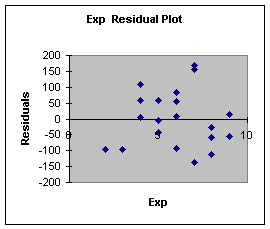Step 7: Use Model for Prediction

If the multiple regression model proves to be statistically and practically useful, and if the assumptions are met, it can then be used for prediction with confidence. I recognize that this model had a problem in that the second independent variable, experience, was determined to be of no statistical utility. The analyst would be better off building a new model without the experience variable, perhaps using some other variable or simply use assets as the single independent variable.

However, I would like to go ahead and make a prediction just to demonstrate the step. As with simple linear regression, we begin by making a point estimate prediction. Let's make our prediction for the first observation in the data set. This prediction involves values of the independent variables as follows. The value for assets is \$3,200,000 and the value for audit team leader experience is 8 years. The point estimate is then:

Eq. 3.1.4: ExtHours = 468.8 + 0.10 (3200) - 2.9 (8) = 765.6

The second step is to make the prediction interval for this observation. We are 95% confident that for a client with \$3,200,000 in assets, and with an audit team leader having 8 years of experience, the audit will take:

Eq. 3.1.5: ExtHours = 765.6 + 2 * standard error of model
ExtHours = 765.6 + 2 * (93.7)

ExtHours = 765.6 + 187.4 or 578.2 to 953.

As a closing note, the actual audit took 700 External Hours for the first observation, which gives an error of -65.6. That error is 9.4% of the actual value.

Excel will produce line fit plots showing the predicted values of Y for all the values of X1 and X2, but produces these as separate two-dimensional plots. It does not have the capability to illustrate the multidimensional surface represented by the multiple regression prediction model.

That ends the introduction. Next, we examine how to incorporate curvature, categorical variables and interaction into the multiple regression model.

Reference:

Anderson, D., Sweeney, D., & Williams, T. (2001). Contemporary Business Statistics with Microsoft Excel. Cincinnati, OH: South-Western, Chapter 12 (Section 12.9).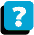About the Course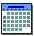Module Schedule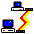WebBoard